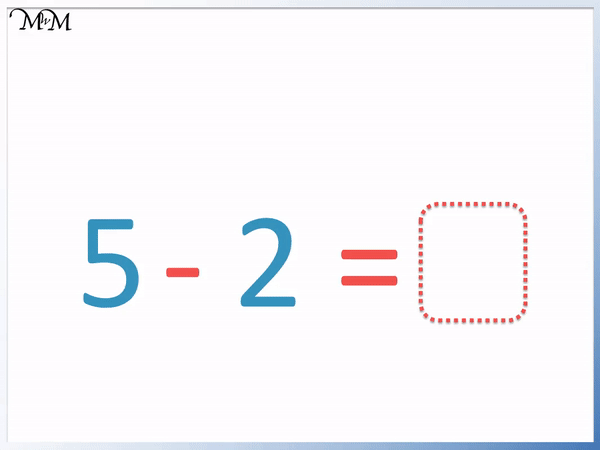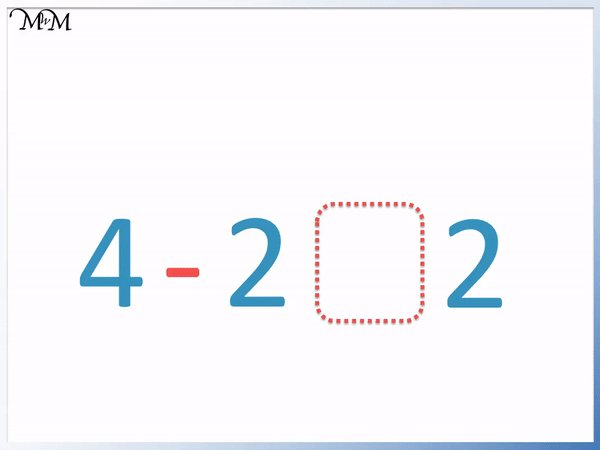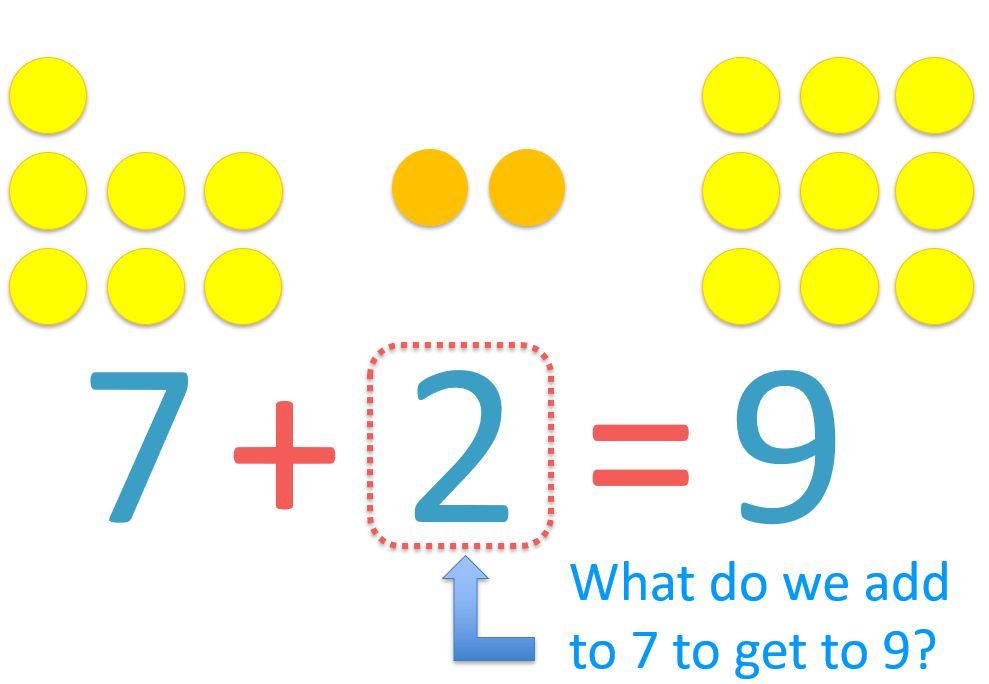# Subtraction Number Sentences

Subtraction Number Sentences• 3 – 1 = 2 is an example of a subtraction number sentence.
• The ‘-‘ sign is called a minus sign or a subtraction sign.
• Subtraction means to remove.
• 3 – 1 means that we start with three counters and remove 1 counter.
• After removing one counter we have 2 counters remaining.
• The = sign is called the equals sign.
• In a number sentence, the equals sign means that the total value on the left of the equals sign is the same as the total value on the right of the equals sign.
• This subtraction number sentence is read as ‘three subtract one equals two’.
• It means that having 3 counters and then removing one counter is the same as simply having 2 counters.
‘-‘ is called the minus sign and it means to take away the number on the right of it from the number on the left of it.

‘=’ is called the equals sign and it means that the values on either side of it are the same.• Here is an example of a subtraction number sentence.
• The subtraction sign ‘-‘ means that we will be removing some counters.
• The number after the subtraction sign is a 2 and so we will remove 2 counters from the 5 counters.
• We are left with 3 counters and so 5 – 2 = 3.
• Our number sentence is 5 – 2 = 3 and is read as ‘five subtract two equals three’.# What are Subtraction Number Sentences?

A number sentence is used to write down a relationship between numbers. They are made up of numbers separated by mathematical symbols, such as ‘+’, ‘-‘ and ‘=’.

A subtraction number sentence will contain the subtraction sign ‘-‘ and and equals sign ‘=’.

In this lesson we will look specifically at subtraction number sentences and learn how to write them.

Below is our first subtraction number sentences example.We will take away one counter as shown.

The word we use for taking away is subtraction. The way to say remove one counter is subtract one.

To write subtract in Maths we use the subtraction sign ‘-‘.

The subtraction sign is also sometimes called a minus sign and it means that we take away the number on its right from the number on its left.

We can write the start of our number sentence as ‘3 – 1’, which is quicker than saying ‘three subtract one’.

We are left with 2 counters remaining after the subtraction of one from three.

In words we could say ‘three subtract one is the same as two’.

The mathematical way to say ‘the same as’ is to say ‘equals’.

The equals sign is ‘=’ and it means that the value on the left is the same as the value on the right.We have our subtraction number sentence written above as 3 – 1 = 2.

If we remove one counter from three original counters we have two remaining and this number sentence tells us that if we started with three counters and then removed one, it is the same as if we simply started with two counters.

Here is another example of a subtraction number sentence.The subtraction sign ‘-‘ comes next and tells us that we will be taking away some counters.

The number of counters that we will be subtracting is the number after the subtraction sign.

There is a ‘2’ after the subtraction sign and so, we will be taking away 2 counters from the original 5.

We are left with three counters and so, after the equals sign ‘=’ we write a 3.

The equals sign tells us that the amount on the left of the ‘=’ sign is the same as the amount on the right of the ‘=’ sign.

Starting with 5 counters but then removing 2 counters results in exactly the same as if we just started with 3 counters.

Here is another subtraction sentence example.Since our missing number is after the equals sign, we want to know which number is the same as 6 – 5.

This means ‘six subtract 5’ and this means start with six counters and take away 5 counters.

We can see that we are left with one counter and so, 1 is the same as 6 – 5.

We write 1 at the end of our subtraction sentence as the answer.

Here is our final subtraction number sentence example and we want to know what symbol to put in the empty box.

We can’t put another number directly next to another number. We need to separate the numbers in our number sentence with mathematical symbols such as ‘+’, ‘-‘ and ‘=’ signs.Here we have 4 counters and are removing 2 counters.

On the right we have 2 counters.

We start the subtraction number sentence with ‘4 – 2’ and so we can work this out.

4 subtract 2 leaves us with 2 counters remaining.

Therefore 4 – 2 is the same as the ‘2’ we have on the right.

To show that 4 – 2 and 2 are the same value, we can put an equals sign in between them.

We have 4 – 2 = 2.

We read this subtraction sentence as ‘four subtract two equals two’.Now try our lesson on Addition Missing Number Problems where we learn how to find missing numbers in addition sentences.